edHelper subscribers - Create a new printable

Number of Pages
 Select the number of pages: 1 page 2 pages 3 pages 4 pages

Answer Key
Include an answer key (answer keys will be at the end of the printable)
No answer key

Not a subscriber?  Sign up now for the subscriber materials!
Sample edHelper.com - Math Review Worksheet
Return to Algebra
Return to Math

 Name _____________________________Date ___________________
Algebra
(Answer ID # 0738396)
Solve each system by graphing. Find the vertices of the graph.
 1 7x + 2y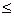-103x - y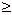-84x + 3y-15
 2 x - 8y-85x + 3y36x - 5y38
 3 2x - y-23x - 5y4x - 4y-1

Factor. One factor is given.
 4 f(x) = x3 + 1Factor: x2 - x + 1
 5 f(x) = -4x3 + 8x2 + 396xFactor: -4x
 6 f(x) = x3 + 9x2 + 15x - 25Factor: x + 5

Solve each equation by factoring.
 7 x2 + 7x - 18 = 0
8.
 -56
x2 -
22
 12
x  =
-8
 13
x2 -
11
 23

9.
 -20x2 + 55x - 35 = 0

Point M is the midpoint between the points P and Q. Find the coordinates of the missing point.
10.
P
 ( 9 , 11 )

M
(6  ,
4
 12
)

11.
Q
 ( 21 , -26 )

M
(
14
 12
,
-17
 12
)

Solve each system of equations using any method.
 12 17x + 10y = 17921x + 10y = 127
 13 13x + 18y = 1123x - 5y = 154

Evaluate the expression.
14.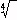144i8d7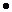224i7d7b12

15.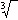11c7405c11384c10

Solve by factoring.
 16 x2 + 16x + 64 = 0
 17 x2 + 16x + 48 = 0
 18 9x2 - 66x + 121 = 0

Use determinants to calculate the area. Show your work.
 19 Calculate the area of the triangle with the following points:(-4, -10), (1, -3), (-2, -5)
 20 Calculate the area of the triangle with the following points:(-5, 0), (0, -4), (4, 5)
 21 Calculate the area of the triangle with the following points:(-4, 2), (-1, 0), (3, 8)

 Answer Key

Sample
This is only a sample worksheet.

edHelper subscribers - Create a new printable

Number of Pages
 Select the number of pages: 1 page 2 pages 3 pages 4 pages

Answer Key
Include an answer key (answer keys will be at the end of the printable)
No answer key

Not a subscriber?  Sign up now for the subscriber materials!
Sample edHelper.com - Math Review Worksheet
Return to Algebra
Return to Math